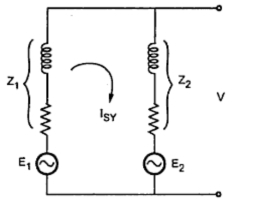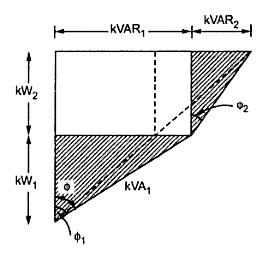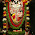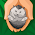Effect of change in Excitation

In case of alternators a field rheostat may be used to change the excitation or its field current. If alternators are running in parallel, a change in the field current will not change the active power shared significantly but will change the operating power factor. With change in the excitation the armature current will change which will change the active power by a small amount. Let us consider the effect of change in excitation on alternator with and without load.
1.1 Alternator on No Load
Consider two alternators on no load and working in parallel. If their excitations are adjusted properly then the e.m.f.s E1 and E2 will be equal. Thus will not be any current in local circuit.
Now say excitation of alternator 1 is increased then magnitude of E1 will be more than that of. This will cause resultant voltage Ēr = Ē1 - Ē2 that will appear in the local circuit. This can be shown in the phasor diagram shown in the Fig. 1.Fig. 1

This resulting voltage will set up a synchronizing current ISY in the local circuit and since the synchronizing impedances are mainly reactive, this current lags Er by approximately 90o.
For alternator 1, ISY lags behind E1 by 90o. This lagging current will produce demagnetizing effect and will try to reduce the generated e.m.f. Alternately for other alternator,  ISY leads E2 by 90o. There will be leading current which will produce magnetizing effect and the field will be strengthened which will try to increase the generated e.m.f. Thus E1 will be reduced whereas E2 will be increased. Hence the circulating current will try to make the two generated e.m.f.s equal at no load whereas the power angle will remain at zero degrees.Fig. 2
1.2 Alternator on Load
Consider again two alternators running in parallel with each alternator supplying one half of active power and one half of reactive power. Each alternator supplies a load current of I such that total load current is 2I. It is assumed that E1 =  E2 while the operating power factor is cos Φ and terminal voltage V. The power triangles for both the alternators can be represented as shown in the Fig. 3. where both active and reactive powers divided equally giving apparent power triangles same.Fig. 3
From the circuit diagram it can be seen that ISY current is vectorially added to the load current of alternator no 2. Now the load currents will be changed to I1 and I2 with change in power factors. The new power factor are cosΦ1 and cosΦ2. This is shown in the Fig. 4.Fig. 4
It can be seen that from the Fig. 4 that cosΦ1 is reduced whereas cosΦ2 is increased. The armature currents for the two machines are changed but their active components are not changed. Thus changes in KW loading of the two alternators is negligible but reactive power KVAR1 from first alternator is increased whereas KVAR2 supplied by second alternator is decreased which can be seen from power triangles.
1.3 Phasor Diagram
The effect of change in excitation on the performance of the alternators can be explained with the help of phasor diagram shown in the Fig.5.Fig. 5
Again the two alternators are working in parallel. If now excitation of alternator 1 is increased so that its induced e.m.f. E1 is increased to E1' which will try to increase the terminal voltage V. But the terminal voltage V can be kept constant by decreasing the excitation of other alternator. The increase in E1 and decrease in E2 are adjusted in such a way that E sin δ remains constant. The difference between E'1 and E'2 give rise to circulating current ISY. This current must be added to I1 and subtracted from I2 which will give new armature currents I'1 and I'2.
It can be seen that there is increase in magnitude of I'1 but its active component I'1 cosΦ1. is unaltered. Similarly I'2 is decreased in magnitude but its active component I'2 cosΦ2. is unaffected. Thus the load current, terminal voltage and load power factors are unchanged.
Form the Fig. 5 it is clear that the alternator 1 operated at a proof p.f. which delivers greater reactive power than alternator 2 operating at a better p.f. Since the mechanical power input to the two alternators is not distributed, the active power output is remaining same. Thus change in excitation causes only the KVAR sharing of the two alternators without distributing kW sharing of the two machines.
Thus the load current, the load terminal voltage and the load power factor remain unchanged but armature currents, induced e.m.f.s and operating power factors remain unchanged but armature currents, induced e.m.f.s and operating power factors for each of the alternator is changed.
Note : By varying the field excitation with the help of rheostats the distribution of reactive power shared by the alternators and their terminal voltage can be controlled.
1.4 Division of Load Between Two Alternators
The division of load between the two alternators can be calculated as follows.Fig. 6
From the Fig. 6 it can be seen that
Substituting the above values in equation 1
From the Fig. 6 it can also be seen that
Subtracting above two equations,
Substituting this value in equation
Note : When two e.m.f.s are unequal in magnitude the second term of above equation represents the circulating current under loaded conditions.
At no load (i.e. Z = ∞) the circulating current is given by,
1.2.